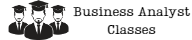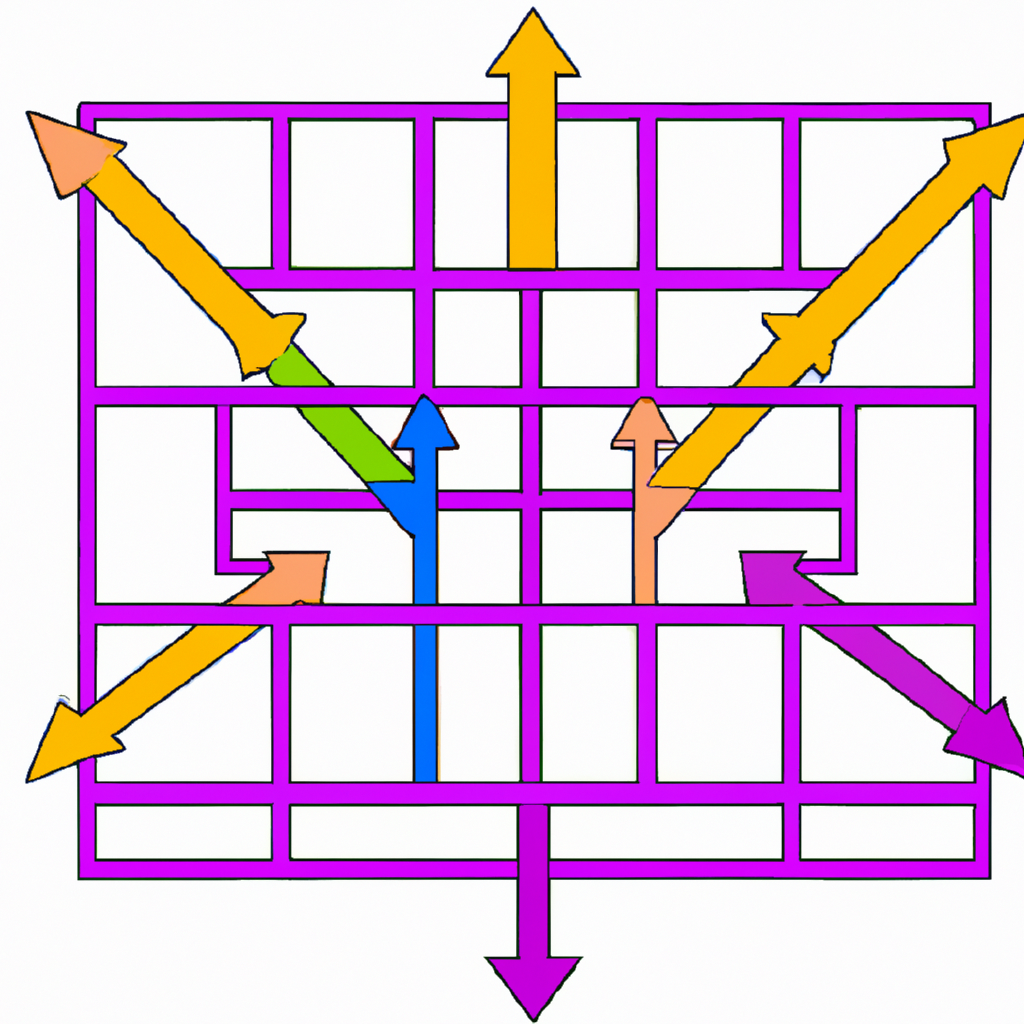Search

# Structural Equation Modeling: Testing Hypotheses and Causal Relationships in Data

Structural equation modeling (SEM) is a statistical technique used to test hypotheses and causal relationships between variables in data. It is a broadly applicable method that can be used to analyze complex systems with multiple interconnected variables. SEM is widely used in social sciences, psychology, marketing research, and other fields, and it is becoming increasingly popular in data science.## What is Structural Equation Modeling?

Structural equation modeling is a statistical technique that allows researchers to test complex relationships between variables. It is a multivariate technique that can be used to analyze data with multiple variables that are thought to be connected in some way. SEM is a flexible method that can be used to test the relationships between latent variables, observed variables, or a combination of both.

SEM is based on the idea of a hypothetical model that represents the relationships between variables in the data. The model is typically represented graphically as a path diagram that shows the relationships between variables. The model is then tested against the data to see if it fits well. If the model fits well, it provides evidence that the relationships depicted in the model are supported by the data.

## The Advantages of Structural Equation Modeling

The advantages of structural equation modeling are numerous. Firstly, SEM can test complex relationships between variables, including direct and indirect effects. Secondly, SEM can handle both continuous and categorical variables. Thirdly, SEM can be used to test the fit of a model to the data, which provides evidence for the validity of the model. Finally, SEM can be used to estimate the parameters of the model, which provides information about the strength and direction of the relationships between variables.

## The Steps Involved in Structural Equation Modeling

There are several steps involved in structural equation modeling. The first step is to specify a theoretical model that represents the relationships between variables in the data. The model should be based on prior research and theory. The second step is to collect data that can be used to test the model. The third step is to estimate the parameters of the model using a statistical software package. The fourth step is to assess the fit of the model to the data. If the model fits well, the final step is to interpret the results and draw conclusions about the relationships between variables.

## The Applications of Structural Equation Modeling

Structural equation modeling has many applications in various fields. It is commonly used in social sciences, psychology, marketing research, and education research. SEM can be used to test theories about the relationships between variables in these fields. For example, SEM can be used to test the relationship between job satisfaction and employee turnover in organizational psychology. It can also be used to test the relationship between marketing strategies and consumer behavior in marketing research.

## The Limitations of Structural Equation Modeling

While structural equation modeling has many advantages, it also has some limitations. Firstly, SEM requires a large sample size to provide reliable results. Secondly, SEM assumes that the data is normally distributed and does not have outliers. Thirdly, SEM can be complex and difficult to understand, especially for those without a strong statistical background. Finally, SEM requires a good understanding of the theory and prior research in the field to develop an appropriate model.

## Conclusion

Structural equation modeling is a valuable tool for testing hypotheses and causal relationships between variables in data. It is a flexible method that can be applied to a wide range of fields and data types. SEM has many advantages, including the ability to test complex relationships, handle both continuous and categorical variables, and estimate parameters. However, it also has limitations, including the need for a large sample size, the assumption of normality and lack of outliers in the data, and the complexity of the method. Overall, structural equation modeling is a powerful statistical technique that can provide valuable insights into the relationships between variables in data.

### Try some of our classes

Enter your email and we’ll send you some samples of our favorite classes.

#### Course Categories

Quisque velit nisi, pretium ut lacinia in, elementum id enim.